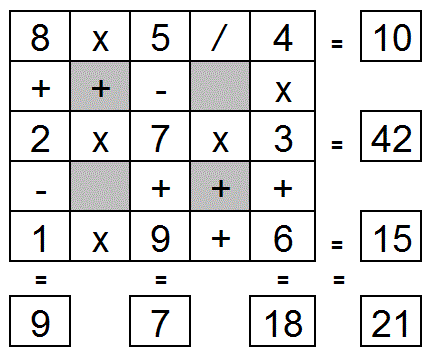Select Page

Each month, a new set of puzzles will be posted.  Come back next month for the solutions and a new set of puzzles, or subscribe to have them sent directly to you.

## MATCHSTICK Challenge

1. Move only two of the matchsticks in diagram 1 to turn the chair upside down.
2. Move only three of the matchsticks in diagram 2 to create three equal squares.

All match sticks must be used.

 12## MIND-Xpander (Circular Logic Challenge – Level 2)

1. You have 100 sticks standing upright in a circle (#1 to #100). Starting with stick #1, knock over the next stick (#2) in the circle. Then continue by knocking over every second stick (next is #4). Keep going around and around the circle until one stick remains standing. What number is the last stick standing?
2. What if you start half way around with stick #50 and continue around and around in same manner as above?

## Feedback

There are more than one way of doing these puzzles and may well be more than one answer.  Please let me and others know what alternatives you find by commenting below.  We also welcome general comments on the subject and any feedback you'd like to give.

If you have a question that needs a response from me or you would like to contact me privately, please use the contact form.

## Get more puzzles!

If you've enjoyed doing the puzzles, consider ordering the books;

• Book One - 150+ of the best puzzles
• Book Two - 200+ with new originals and more of your favourites

Both in a handy pocket sized format.   Click here for full details.

## EQUATE+2 Puzzle

Each row, column & diagonal is an equation and you use the numbers 1 to 9 to complete the equations. Each number can be used only once. ‘Two’ numbers have been provided to get you started. Find the remaining seven numbers that satisfies all the resulting equations. Note – multiplication (x) & division (/) are performed before addition (+) and subtraction (-).

Solution:## EQUATE+0 Puzzle

Each row, column & diagonal is an equation and you use the numbers 1 to 9 to complete the equations. Each number can be used only once. Find the remaining nine numbers that satisfies all the resulting equations. Note – multiplication (x) & division (/) are performed before addition (+) and subtraction (-)

Solution: